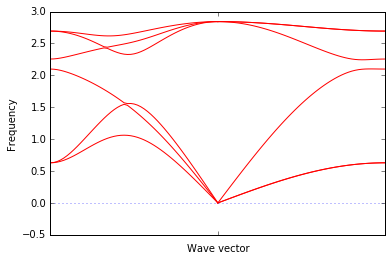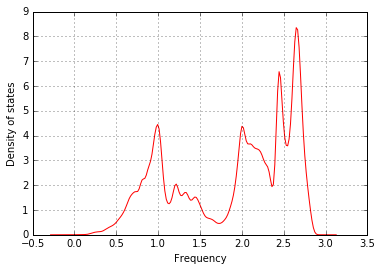This notebook shows how to use the output from VASP DFPT calculation and the phonopy interface to plot the phonon bandstructure and density of states.

Requires: phonopy package (pip install phonopy)

Author: Kiran Mathew

In :
import os
import numpy as np

import pymatgen as pmg
from pymatgen.io.vasp.outputs import Vasprun

from phonopy import Phonopy
from phonopy.structure.atoms import Atoms as PhonopyAtoms

%matplotlib inline


Set the structure

In :
Si_primitive = PhonopyAtoms(symbols=['Si'] * 2,
scaled_positions=[(0, 0, 0), (0.75, 0.5, 0.75)],
cell=[[3.867422 ,0.000000, 0.000000],
[1.933711, 3.349287, 0.000000],
[-0.000000, -2.232856, 3.157737]])

# supercell size
scell = [[2,0,0],[0,2,0],[0,0,2]]


Result from VASP DFPT calculations using the supercell structure

In :
vrun = Vasprun(os.path.join(os.path.dirname(pmg.__file__), "..", 'test_files', "vasprun.xml.dfpt.phonon"))

/home/ray/Software/matproj/pymatgen/pymatgen/io/vasp/outputs.py:814: UserWarning: No POTCAR file with matching TITEL fields was found in /home/ray/Software/matproj/pymatgen/test_files
" was found in {}".format(os.path.abspath(p)))


Initialize phonopy and set the force constants obtained from VASP

In :
phonon = Phonopy(Si_primitive, scell)

# negative sign to ensure consistency with phonopy convention
phonon.set_force_constants(-vrun.force_constants)


Define the paths for plotting the bandstructure and set them in phonopy

In :
bands = []

# path 1
q_start  = np.array([0.5, 0.5, 0.0])
q_end    = np.array([0.0, 0.0, 0.0])
band = []
for i in range(51):
band.append(q_start + (q_end - q_start) / 50 * i)
bands.append(band)

# path 2
q_start  = np.array([0.0, 0.0, 0.0])
q_end    = np.array([0.5, 0.0, 0.0])
band = []
for i in range(51):
band.append(q_start + (q_end - q_start) / 50 * i)
bands.append(band)

phonon.set_band_structure(bands)

Out:
True
In :
phonon.plot_band_structure().show()Set the mesh in reciprocal space and plot DOS

In :
mesh = [31, 31, 31]
phonon.set_mesh(mesh)

phonon.set_total_DOS()
phonon.plot_total_DOS().show()